# fillmissing

Fill missing entries

## Syntax

``F = fillmissing(A,'constant',v)``
``F = fillmissing(A,method)``
``F = fillmissing(A,movmethod,window)``
``F = fillmissing(A,'knn')``
``F = fillmissing(A,'knn',k)``
``F = fillmissing(A,fillfun,gapwindow)``
``F = fillmissing(___,dim)``
``F = fillmissing(___,Name,Value)``
``````[F,TF] = fillmissing(___)``````

## Description

example

````F = fillmissing(A,'constant',v)` fills missing entries of an array or table with the constant value `v`. If `A` is a matrix or multidimensional array, then `v` can be either a scalar or a vector. If `v` is a vector, then each element specifies the fill value in the corresponding column of `A`. If `A` is a table or timetable, then `v` can also be a cell array whose elements contain fill values for each table variable.Missing values are defined according to the data type of `A`: `NaN` — `double`, `single`, `duration`, and `calendarDuration``NaT` — `datetime``<missing>` — `string``<undefined>` — `categorical``{''}` — `cell` of character vectors If `A` is a table, then the data type of each variable defines the missing value for that variable.```

example

````F = fillmissing(A,method)` fills missing entries using the method specified by `method`. For example, `fillmissing(A,'previous')` fills missing entries with the previous nonmissing entry of `A`.```

example

````F = fillmissing(A,movmethod,window)` fills missing entries using a moving window mean or median with window length `window`. For example, `fillmissing(A,'movmean',5)` fills data with a moving mean using a window length of 5.```

example

````F = fillmissing(A,'knn')` fills missing entries with the corresponding values from the nearest neighbor rows, calculated based on the pairwise Euclidean distance between rows.```
````F = fillmissing(A,'knn',k)` fills missing entries with the mean of the corresponding values from the `k` nearest neighbor rows, calculated based on the pairwise Euclidean distance between rows. For example, `fillmissing(A,'knn',5)` fills missing entries of `A` with the mean of the corresponding values from the five nearest neighbor rows.```

example

````F = fillmissing(A,fillfun,gapwindow)` fills gaps of missing entries using a custom method specified by a function handle `fillfun` and a fixed window surrounding each gap from which the fill values are computed. `fillfun` must have the input arguments `xs`, `ts`, and `tq`, which are vectors containing the sample data `xs` of length `gapwindow`, the sample data locations `ts` of length `gapwindow`, and the missing data locations `tq`. The locations in `ts` and `tq` are a subset of the sample points vector.```

example

````F = fillmissing(___,dim)` specifies the dimension of `A` to operate along in addition to any of the input argument combinations in previous syntaxes. By default, `fillmissing` operates along the first dimension whose size does not equal 1. For example, if `A` is a matrix, then `fillmissing(A,2)` operates across the columns of `A`, filling missing data row by row.```

example

````F = fillmissing(___,Name,Value)` specifies additional parameters for filling missing values using one or more name-value arguments. For example, if `t` is a vector of time values, then `fillmissing(A,'linear','SamplePoints',t)` interpolates the data in `A` relative to the times in `t`.```

example

``````[F,TF] = fillmissing(___)``` also returns a logical array `TF` that indicates the position of the filled entries in `F` that were previously missing.```

## Examples

collapse all

Create a vector that contains `NaN` values, and replace each `NaN` with the previous nonmissing value.

```A = [1 3 NaN 4 NaN NaN 5]; F = fillmissing(A,'previous')```
```F = 1×7 1 3 3 4 4 4 5 ```

Create a 2-by-2 matrix with a `NaN` value in each column. Fill `NaN` with `100` in the first column and `1000` in the second column.

`A = [1 NaN; NaN 2]`
```A = 2×2 1 NaN NaN 2 ```
`F = fillmissing(A,'constant',[100 1000])`
```F = 2×2 1 1000 100 2 ```

Use interpolation to replace `NaN` values in nonuniformly sampled data.

Define a vector of nonuniform sample points and evaluate the sine function over the points.

```x = [-4*pi:0.1:0, 0.1:0.2:4*pi]; A = sin(x);```

Inject `NaN` values into `A`.

`A(A < 0.75 & A > 0.5) = NaN;`

Fill the missing data using linear interpolation, and return the filled vector `F` and the logical vector `TF`. The value 1 (`true`) in entries of `TF` corresponds to the values of `F` that were filled.

`[F,TF] = fillmissing(A,'linear','SamplePoints',x);`

Plot the original data and filled data.

```scatter(x,A) hold on scatter(x(TF),F(TF)) legend('Original Data','Filled Data')```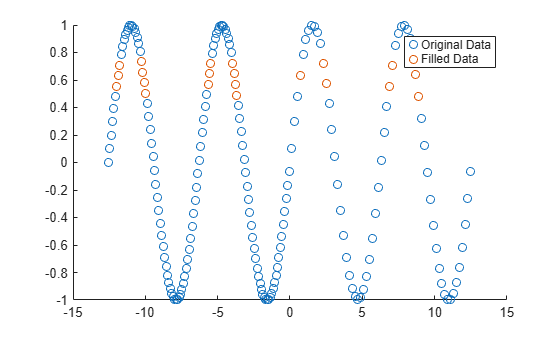Use a moving median to fill missing numeric data.

Create a vector of sample points `x` and a vector of data `A` that contains missing values.

```x = linspace(0,10,200); A = sin(x) + 0.5*(rand(size(x))-0.5); A([1:10 randi([1 length(x)],1,50)]) = NaN; ```

Replace `NaN` values in `A` using a moving median with a window of length 10, and plot the original data and the filled data.

```F = fillmissing(A,'movmedian',10); plot(x,F,'.-') hold on plot(x,A,'.-') legend('Original Data','Filled Data')```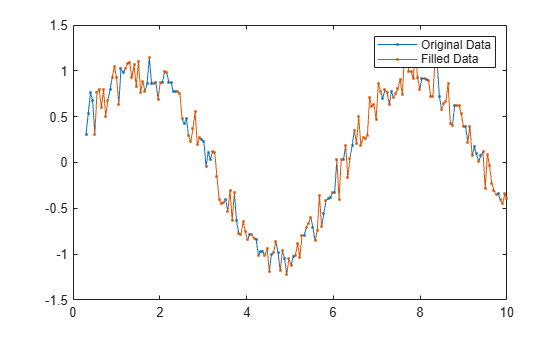Define a custom function to fill `NaN` values with the previous nonmissing value.

Define a vector of sample points `t` and a vector of corresponding data `A` containing `NaN` values. Plot the data.

```t = 10:10:100; A = [0.1 0.2 0.3 NaN NaN 0.6 0.7 NaN 0.9 1]; scatter(t,A)```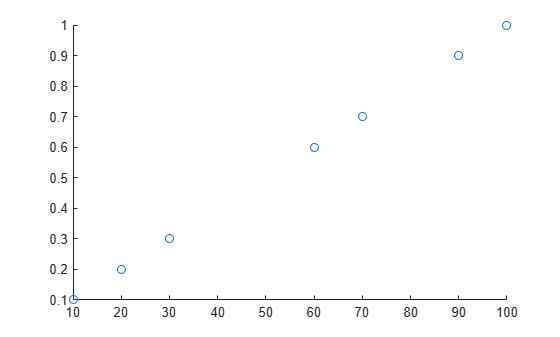Use the local function `forwardfill` (defined at the end of the example) to fill missing gaps with the previous nonmissing value. The function handle inputs include:

• `xs` — data values used for filling

• `ts` — locations of the values used for filling relative to the sample points

• `tq` — locations of the missing values relative to the sample points

• `n` — number of values in the gap to fill

```n = 2; gapwindow = [10 0]; [F,TF] = fillmissing(A,@(xs,ts,tq) forwardfill(xs,ts,tq,n),gapwindow,'SamplePoints',t);```

The gap window value `[10 0]` tells `fillmissing` to consider one data point before a missing gap and no data points after a gap, since the previous nonmissing value is located 10 units prior to the gap. The function handle input values determined by `fillmissing` for the first gap are:

• `xs = 0.3`

• `ts = 30`

• `tq = [40 50]`

The function handle input values for the second gap are:

• `xs = 0.7`

• `ts = 70`

• `tq = 80`

Plot the original data and the filled data.

```scatter(t,A) hold on scatter(t(TF),F(TF))```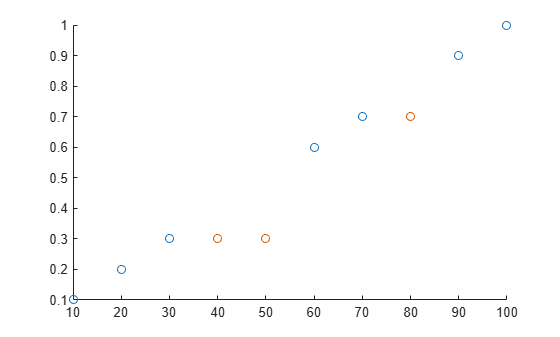```function y = forwardfill(xs,ts,tq,n) % Fill n values in the missing gap using the previous nonmissing value y = NaN(1,numel(tq)); y(1:min(numel(tq),n)) = xs; end```

Create a matrix with missing entries and fill across the columns (second dimension) one row at a time using linear interpolation. For each row, fill leading and trailing missing values with the nearest nonmissing value in that row.

```A = [NaN NaN 5 3 NaN 5 7 NaN 9 NaN; 8 9 NaN 1 4 5 NaN 5 NaN 5; NaN 4 9 8 7 2 4 1 1 NaN]```
```A = 3×10 NaN NaN 5 3 NaN 5 7 NaN 9 NaN 8 9 NaN 1 4 5 NaN 5 NaN 5 NaN 4 9 8 7 2 4 1 1 NaN ```
`F = fillmissing(A,'linear',2,'EndValues','nearest')`
```F = 3×10 5 5 5 3 4 5 7 8 9 9 8 9 5 1 4 5 5 5 5 5 4 4 9 8 7 2 4 1 1 1 ```

Fill missing values for table variables with different data types.

Create a table whose variables include `categorical`, `double`, and `char` data types.

```A = table(categorical({'Sunny'; 'Cloudy'; ''}),[66; NaN; 54],{''; 'N'; 'Y'},[37; 39; NaN],... 'VariableNames',{'Description' 'Temperature' 'Rain' 'Humidity'})```
```A=3×4 table Description Temperature Rain Humidity ___________ ___________ __________ ________ Sunny 66 {0x0 char} 37 Cloudy NaN {'N' } 39 <undefined> 54 {'Y' } NaN ```

Replace all missing entries with the value from the previous entry. Since there is no previous element in the `Rain` variable, the missing character vector is not replaced.

`F = fillmissing(A,'previous')`
```F=3×4 table Description Temperature Rain Humidity ___________ ___________ __________ ________ Sunny 66 {0x0 char} 37 Cloudy 66 {'N' } 39 Cloudy 54 {'Y' } 39 ```

Replace the `NaN` values from the `Temperature` and `Humidity` variables in `A` with 0.

`F = fillmissing(A,'constant',0,'DataVariables',{'Temperature','Humidity'})`
```F=3×4 table Description Temperature Rain Humidity ___________ ___________ __________ ________ Sunny 66 {0x0 char} 37 Cloudy 0 {'N' } 39 <undefined> 54 {'Y' } 0 ```

Alternatively, use the `isnumeric` function to identify the numeric variables to operate on.

`F = fillmissing(A,'constant',0,'DataVariables',@isnumeric)`
```F=3×4 table Description Temperature Rain Humidity ___________ ___________ __________ ________ Sunny 66 {0x0 char} 37 Cloudy 0 {'N' } 39 <undefined> 54 {'Y' } 0 ```

Now fill the missing values in `A` with a specified constant for each table variable, which are contained in a cell array.

`F = fillmissing(A,'constant',{categorical({'None'}),1000,'Unknown',1000})`
```F=3×4 table Description Temperature Rain Humidity ___________ ___________ ___________ ________ Sunny 66 {'Unknown'} 37 Cloudy 1000 {'N' } 39 None 54 {'Y' } 1000 ```

Create a time vector `t` in seconds and a corresponding vector of data `A` that contains `NaN` values.

```t = seconds([2 4 8 17 98 134 256 311 1001]); A = [1 3 23 NaN NaN NaN 100 NaN 233];```

Fill only missing values in `A` that correspond to a maximum gap size of 250 seconds. Because the second gap is larger than 250 seconds, the `NaN` value is not filled.

`F = fillmissing(A,'linear','SamplePoints',t,'MaxGap',seconds(250))`
```F = 1×9 1.0000 3.0000 23.0000 25.7944 50.9435 62.1210 100.0000 NaN 233.0000 ```

Use custom distance functions to fill missing entries using values from nearest neighbor rows.

Create a matrix that contains a `NaN` value, and then create a logical vector that indicates the locations of missing entries in the third row.

```A = [1 3 9 3; -5 1 7 2; -1 1 7 NaN; 12 1 9 1]; m = isnan(A(3,:));```

Define two custom functions to measure distances between rows.

The function `d1` measures distances between rows by summing up the distances between each coordinate pair; the function `dinf` measures distances between rows by finding the maximum distance among the coordinate pairs.

```d1 = @(x,~) sum(abs(diff(x)),'omitnan'); dinf = @(x,isNaN) norm(diff(x(:,~isNaN(1,:))),'inf');```

Compute the `d1`-measured distance between the third row and each of the other three rows. The second row is the closest.

`d1s = arrayfun(@(r) d1(A([r 3],:),m), setdiff(1:4,3))`
```d1s = 1×3 6 4 15 ```

The `fillmissing` function replaces the `NaN` in the third row with the corresponding `2` from the second row.

`F1 = fillmissing(A,'knn','Distance',d1)`
```F1 = 4×4 1 3 9 3 -5 1 7 2 -1 1 7 2 12 1 9 1 ```

A similar analysis with `dinf`-measured distances finds the first row to be closest to the third. Now the `fillmissing` function replaces the `NaN` in the third row with the corresponding 3 from the first row.

`dinfs = arrayfun(@(r) dinf(A([r 3],:),m), setdiff(1:4,3))`
```dinfs = 1×3 2 4 13 ```
`Finf = fillmissing(A,'knn','Distance',dinf)`
```Finf = 4×4 1 3 9 3 -5 1 7 2 -1 1 7 3 12 1 9 1 ```

## Input Arguments

collapse all

Input data, specified as a vector, matrix, multidimensional array, cell array of character vectors, table, or timetable.

• If `A` is a timetable, then only table values are filled. If the associated vector of row times contains a `NaT` or `NaN` value, then `fillmissing` produces an error. Row times must be unique and listed in ascending order.

• If `A` is a cell array or a table with cell array variables, then `fillmissing` only fills missing elements when the cell array contains character vectors.

Fill constant, specified as a scalar, vector, or cell array.

• If `A` is a matrix or multidimensional array, then `v` can be a vector indicating a different fill value for each operating dimension. The length of `v` must match the length of the operating dimension.

• If `A` is a table or timetable, then `v` can be a cell array of fill values indicating a different fill value for each variable. The number of elements in the cell array must match the number of variables in the table.

Fill method, specified as one of these values:

MethodDescription
`'previous'`Previous nonmissing value
`'next'`Next nonmissing value
`'nearest'`Nearest nonmissing value
`'linear'`Linear interpolation of neighboring, nonmissing values (numeric, `duration`, and `datetime` data types only)
`'spline'`Piecewise cubic spline interpolation (numeric, `duration`, and `datetime` data types only)
`'pchip'`Shape-preserving piecewise cubic spline interpolation (numeric, `duration`, and `datetime` data types only)
`'makima'`Modified Akima cubic Hermite interpolation (numeric, `duration`, and `datetime` data types only)

Moving method to fill missing data, specified as one of these values:

MethodDescription
`'movmean'`Moving average over a window of length `window` (numeric data types only)
`'movmedian'`Moving median over a window of length `window` (numeric data types only)

Window length for moving methods, specified as a positive integer scalar, a two-element vector of positive integers, a positive duration scalar, or a two-element vector of positive durations. The window is defined relative to the sample points.

If `window` is a positive integer scalar, then the window is centered about the current element and contains `window-1` neighboring elements. If `window` is even, then the window is centered about the current and previous elements.

If `window` is a two-element vector of positive integers `[b f]`, then the window contains the current element, `b` elements backward, and `f` elements forward.

If `A` is a timetable or `SamplePoints` is specified as a `datetime` or `duration` vector, then the window must be of type `duration`.

Number of nearest neighbors to average with the `'knn'` method, specified as a positive integer scalar.

Example: `@(xs,ts,tq) myfun(xs,ts,tq)`

Custom fill method, specified as a function handle. Valid function handles must include the following three input arguments:

Input ArgumentDescription
`xs`Vector containing data values used for filling. The length of `xs` must match the length of the specified window.
`ts`Vector containing locations of the values used for filling. The length of `ts` must match the length of the specified window. `ts` is a subset of the sample points vector.
`tq`Vector containing locations of the missing values. `tq` is a subset of the sample points vector.

The function must return either a scalar or a vector with the same length as `tq`.

Gap window length for custom fill functions, specified as a positive integer scalar, a two-element vector of positive integers, a positive duration scalar, or a two-element vector of positive durations. The gap window is defined relative to the sample points.

When specifying a function handle `fillfun` for the fill method, the value of `gapwindow` represents a fixed window length that surrounds each gap of missing values in the input data. The fill value is then computed by `fillfun` using the values in that window. For example, for default sample points `t = 1:10` and data ```A = [10 20 NaN NaN 50 60 70 NaN 90 100]```, a window length `gapwindow = 3` specifies the first gap window as `[20 NaN NaN 50]` that `fillfun` operates on to compute the fill value. The second gap window that `fillfun` operates on is `[70 NaN 90]`.

When `A` is a timetable or `SamplePoints` is specified as a `datetime` or `duration` vector, `gapwindow` must be of type `duration`.

Operating dimension, specified as a positive integer scalar. If no value is specified, then the default is the first array dimension whose size does not equal 1.

Consider an `m`-by-`n` input matrix, `A`:

• `fillmissing(A,method,1)` fills missing values according to the data in each column of `A` and returns an `m`-by-`n` matrix.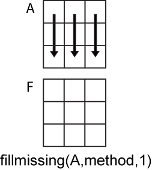• `fillmissing(A,method,2)` fills missing values according to the data in each row of `A` and returns an `m`-by-`n` matrix.For table or timetable input data, `dim` is not supported and operation is along each table or timetable variable separately.

### Name-Value Arguments

Specify optional pairs of arguments as `Name1=Value1,...,NameN=ValueN`, where `Name` is the argument name and `Value` is the corresponding value. Name-value arguments must appear after other arguments, but the order of the pairs does not matter.

Before R2021a, use commas to separate each name and value, and enclose `Name` in quotes.

Example: `fillmissing(T,method,'SamplePoints',"Var1")`

Data Options

collapse all

Sample points, specified as a vector of sample point values or one of the options in the following table when the input data is a table. The sample points represent the x-axis locations of the data, and must be sorted and contain unique elements. Sample points do not need to be uniformly sampled. The vector `[1 2 3 ...]` is the default.

When the input data is a table, you can specify the sample points as a table variable using one of these options:

Indexing SchemeExamples

Variable name:

• A string scalar or character vector

• `"A"` or `'A'` — A variable named `A`

Variable index:

• An index number that refers to the location of a variable in the table

• A logical vector. Typically, this vector is the same length as the number of variables, but you can omit trailing `0` or `false` values

• `3` — The third variable from the table

• `[false false true]` — The third variable

Function handle:

• A function handle that takes a table variable as input and returns a logical scalar

• `@isnumeric` — One variable containing numeric values

Variable type:

• `vartype("numeric")` — One variable containing numeric values

Note

This name-value argument is not supported when the input data is a `timetable`. Timetables use the vector of row times as the sample points. To use different sample points, you must edit the timetable so that the row times contain the desired sample points.

Moving windows are defined relative to the sample points. For example, if `t` is a vector of times corresponding to the input data, then `fillmissing(rand(1,10),'movmean',3,'SamplePoints',t)` has a window that represents the time interval between `t(i)-1.5` and `t(i)+1.5`.

When the sample points vector has data type `datetime` or `duration`, the moving window length must have type `duration`.

Example: ```fillmissing([1 NaN 3 4],'linear','SamplePoints',[1 2.5 3 4])```

Example: `fillmissing(T,'linear','SamplePoints',"Var1")`

Data Types: `single` | `double` | `datetime` | `duration`

Table variables to operate on, specified as one of the options in this table. The `DataVariables` value indicates which variables of the input table to fill.

Other variables in the table not specified by `DataVariables` pass through to the output without being filled.

Indexing SchemeExamples

Variable names:

• A string, character vector, or cell array

• A `pattern` object

• `"A"` or `'A'` — A variable named `A`

• `["A","B"]` or `{'A','B'}` — Two variables named `A` and `B`

• `"Var"+digitsPattern(1)` — Variables named `"Var"` followed by a single digit

Variable index:

• An index number that refers to the location of a variable in the table

• A vector of numbers

• A logical vector. Typically, this vector is the same length as the number of variables, but you can omit trailing `0` or `false` values

• `3` — The third variable from the table

• `[2 3]` — The second and third variables from the table

• `[false false true]` — The third variable

Function handle:

• A function handle that takes a table variable as input and returns a logical scalar

• `@isnumeric` — All the variables containing numeric values

Variable type:

• `vartype("numeric")` — All the variables containing numeric values

Example: ```fillmissing(T,'linear','DataVariables',["Var1" "Var2" "Var4"])```

Replace values indicator, specified as one of these values when `A` is a table or timetable:

• `true` or `1` — Replace input table variables containing missing entries with filled table variables.

• `false` or `0` — Append the input table with all table variables that were checked for missing entries. The missing entries in the appended variables are filled.

For vector, matrix, or multidimensional array input data, `ReplaceValues` is not supported.

Example: `fillmissing(T,'previous','ReplaceValues',false)`

Missing Value Options

collapse all

Method for handling endpoints, specified as `'extrap'`, `'previous'`, `'next'`, `'nearest'`, `'none'`, or a constant scalar value. The endpoint fill method handles leading and trailing missing values based on these definitions:

MethodDescription
`'extrap'`Same as `method`
`'previous'`Previous nonmissing value
`'next'`Next nonmissing value
`'nearest'`Nearest nonmissing value
`'none'`No fill value
scalarConstant value (numeric, `duration`, and `datetime` data types only)

Known missing indicator, specified as a logical vector, matrix, or multidimensional array of the same size as `A`. The indicator elements can be `1` (`true`) to indicate a missing value in the corresponding location of `A` or `0` (`false`) otherwise.

Data Types: `logical`

Maximum gap size to fill, specified as a numeric scalar, `duration` scalar, or `calendarDuration` scalar. Gaps are clusters of consecutive missing values whose size is the distance between the nonmissing values surrounding the gap. The gap size is computed relative to the sample points. Gaps smaller than or equal to the max gap size are filled, and gaps larger than the gap size are not.

For example, consider the vector `y = [25 NaN NaN 100]` using the default sample points `[1 2 3 4]`. The gap size in the vector is computed from the sample points as `4 - 1 = 3`, so a `MaxGap` value of `2` leaves the missing values unaltered, while a `MaxGap` value of `3` fills in the missing values.

For missing values at the beginning or end of the data:

• A single missing value at the beginning or at the end of the input data has a gap size of 0 and is always filled.

• Clusters of missing values occurring at the beginning or end of the input data are not completely surrounded by nonmissing values, so the gap size is computed using the nearest existing sample points. For the default sample points `1:N`, this produces a gap size that is 1 smaller than if the same cluster occurred in the middle of the data.

Distance function to use when finding nearest neighbor rows, specified as `'euclidean'` (Euclidean distance), `'seuclidean'` (scaled Euclidean distance), or a function handle for a distance function.

If you specify a function handle for a distance function, the function must satisfy these conditions:

• The function must accept two inputs.

• The first input of the function must be a two-row matrix, table, or timetable that contains the two vectors to be compared.

• The second input of the function must be a logical matrix that indicates the locations of missing values in the vectors. You can ignore the second input by specifying it as `~`.

• The function must return the distance as a real, scalar value of type `double`.

Example: ```fillmissing(A,'knn','Distance',@(x,~) sum(abs(diff(x)),'omitmissing'))```

## Output Arguments

collapse all

Filled data, returned as a vector, matrix, multidimensional array, table, or timetable.

`F` is the same size as `A` unless the value of `ReplaceValues` is `false`. If the value of `ReplaceValues` is `false`, then the width of `F` is the sum of the input data width and the number of data variables specified.

Filled data indicator, returned as a vector, matrix, or multidimensional array. `TF` is a logical array where `1` (`true`) corresponds to filled entries in `F` that were previously missing and `0` (`false`) corresponds to unchanged entries.

`TF` is the same size as `F`.

Data Types: `logical`

## Tips

• For input data that is a structure array or a cell array of non-character vectors, `fillmissing` does not fill any entries. To fill missing entries in a structure array, apply `fillmissing` to each field in the structure by using the `structfun` function. To fill missing entries in a cell array of non-character vectors, apply `fillmissing` to each cell in the cell array by using the `cellfun` function.

## Version History

Introduced in R2016b

expand all